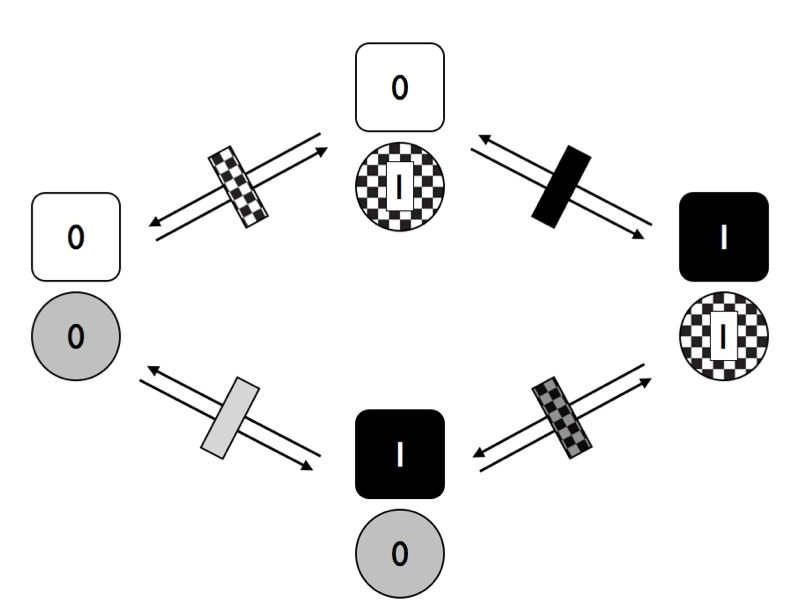9.7: Testing for non-independent evolution of different characters

Hypotheses in evolutionary biology often relate to whether two (or more) traits affect the evolution of one another (Chapter 5). One can have a standard correlation between two discrete traits if knowing the state of one trait allows you to predict the state of the other. However, in evolution, these correlations will arise due to the shared patterns of relatedness across species. We are typically more interested in evolutionary correlations (Chapter 5). With discrete traits, we can define evolutionary correlations in a specific way: two discrete traits share an evolutionary correlation if the state of one character affects the relative transition rates of a second.

Imagine that we are considering the evolution of two traits, trait 1 and trait 2, on a phylogenetic tree. Both traits have two possible character states, one and zero. We can show these two traits visually as Figure 9.4.Figure 9.4. Two discrete character traits, each with two states (labeled 0 and 1). Image by the author, can be reused under a CC-BY-4.0 license.

In the figure, each trait has two possible transition rates, from 0 to 1 and from 1 to 0. For now, let’s assume that backwards and forward rates are equal. Any species can have one of four possible combinations of the two traits (00, 01, 10, or 11). We can draw the transitions among these four combinations as Figure 9.5.Figure 9.5. Transitions among states for two traits with two character states each where characters evolve independently of one another. Image by the author, can be reused under a CC-BY-4.0 license.

In Figure 9.6, I have marked the distinct rates with different rectangles – black represents changes in trait 1, while checkered is changes in trait 2. Notice that, in this figure, we are assuming that the two traits are independent. That is, in this model the transition rates of trait one do not depend on the state of trait 2, and vice-versa. What would happen to our model if we allow the traits to evolve in a dependent manner?Figure 9.6. Transitions among states for two traits with two character states each where characters evolve at rates that depend on the character state of the other trait. Image by the author, can be reused under a CC-BY-4.0 license.

Notice that in Figure 9.6, we have four different transition rates. Consider first the solid rectangles. The grey rectangle represents the transition rate for trait 1 when trait 2 has state 0, while the black rectangle represents the transition rate for trait 1 when trait 2 has state 1. If these two rates are different, then the traits are dependent on each other – that is, the rate of evolution of trait 1 depends on the character state of trait 2.

These two models have different numbers of parameters, but are relatively easy to fit using the maximum-likelihood approach outlined in this chapter. The key is to write down the transition matrix (Q) for each model. For example, a transition matrix for model in figure 9.4 is:

$$\mathbf{Q} = \begin{bmatrix} -q_1 - q_2 & q_1 & q_2 & 0\\ q_1 & -q_1 - q_2 & 0 & q_2\\ q_2 & 0 & -q_1 - q_2 & q_1\\ 0 & q_2 & q_1 & -q_1 - q_2\\ \end{bmatrix} \label{9.2}$$

In the matrix above, each row and column corresponds to a particular combination of states for character 1 and 2: (0,0), (0,1), (1,0), and (1,1). Note that some possible transitions in this model have rate 0, meaning they do not occur. These are transitions that would require both characters to change exactly simultaneously (e.g. (0,0) to (1,1) – a possibility that is excluded from this model.

Similarly, we can write a transition matrix for the model in figure 9.5:

$$\mathbf{Q} = \begin{bmatrix} -q_1 - q_2 & q_1 & q_2 & 0\\ q_1 & -q_1 - q_3 & 0 & q_3\\ q_2 & 0 & -q_2 - q_4 & q_4\\ 0 & q_3 & q_4 & -q_3 - q_4\\ \end{bmatrix} \label{9.3}$$

Notice that the simple, 2-parameter independent evolution model is a special case of the more complex, 4-parameter dependent model. Because of this, we can compare the two with a likelihood ratio test. Alternatively, AIC or Bayes factors can be used. If we find support for the 4-parameter model, we can conclude that the evolution of at least one of the two characters depends on the state of the other.

It is worth noting that there are other models that one can fit for the evolution of two binary traits that I did not discuss above. For example, one can model the situation where the two traits each have different forwards and backwards rates, but are evolving independently. This is a four-parameter model. Additionally, one can allow both forward and backward rates to differ and to depend on the character state of the other trait: an eight-parameter model. This is the model one needs to truly see a correlation between the two characters, one where certain combinations tend to accumulate in the tree. All of these models – and others not described here – can be compared using AIC, BIC, or Bayes Factors. Pagel and Meade (2006) describe a particularly innovative and synthetic method to test hypotheses about correlated evolution of discrete characters in a Bayesian framework using reversible-jump MCMC.

One can also test for correlations among discrete characters using threshold models. Here, one tests whether or not the liabilities for the two characters evolve in a correlated fashion. More specifically, we can model liabilities for the two threshold characters using a bivariate Brownian motion model, with some evolutionary covariance σ122 between the two liabilities. We can then use either ML or Bayesian methods to determine if the evolutionary covariance between the two characters is non-zero (following the methods described in chapter 5, but using likelihoods based on discrete characters as described above).